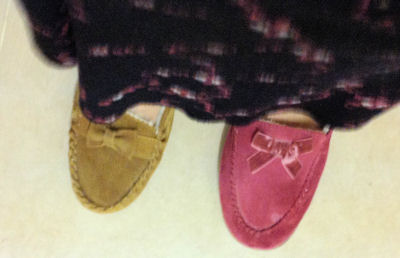#Without a calculator copy and complete:

$$4\times4=16$$
$$3\times3 = 9$$
$$2\times2 =$$
$$1\times1 =$$
$$0\times0 =$$
$$-1\times-1 =$$
$$-2\times$$
$$-3\times$$
$$-4\times$$
$$-5\times$$

If a = 6, b = -6 and c = -6
find the values of:

$$a + b$$
$$ac$$
$$b - c$$
$$abc$$
$$a + b + c$$
$$bc^2$$
$$(bc)^2$$
$$a^2b^3$$
$$c(b - a)$$
$$b^a$$

## A Mathematics Lesson Starter Of The Day

Share

Topics: Starter | Negative Numbers | Number

• Transum,
•
• Have you ever tried to explain to pupils why a negative number multiplied by a negative number gives a positive result? It is difficult to find a real world example that makes this concept clear. My favourite method is to use the pattern or sequence generated in the left frame above. Most pupils take this as a good explanation for the product of negatives result. The right frame above is simply a chance to put this knowledge into practice with some directed number questions.
• Stafford,
•
• A negative x a negative:
The way I explain it to the kids if they're struggling to get it is to ignore the minus signs and do the multiplication. So -5 x -8 do as 5x8=40. If you then put 1 minus on the answer it becomes -40. But we have 2 minuses to include so it'll be 40 and we know that a minus and a minus together make a + (I always do adding/subtracting before multiplying/dividing).
• RER, Paris
•
• Great resource, however some of the questions on the left hand side are incomplete eg 0x then there is no other number. Perhaps this could be amended. The same is true if you ask for different numbers.

[Transum: Thank you for your comments. The left column is intended to be an unfinished sequence of calculations that the pupils should complete. By seeing the patterns in the sequence the pupils might gain a better understanding of directed number.]
• Transum,
•
• My octogenarian mother put on odd slippers today. She thought that was a very negative thing to do. If her slippers were the same it would be a more positive thing!If you remember that you'll have a good way of remembering what happens for division and multiplication. If the numbers have different signs (one positive and the other negative) then the result will be negative. If the signs are the same (either both positive or both negative) the result will be positive.

This notion is for multiplying and dividing only. For adding and subtracting directed numbers refer to the Number Line.

How did you use this starter? Can you suggest how teachers could present or develop this resource? Do you have any comments? It is always useful to receive feedback and helps make this free resource even more useful for Maths teachers anywhere in the world.

If you don't have the time to provide feedback we'd really appreciate it if you could give this page a score! We are constantly improving and adding to these starters so it would be really helpful to know which ones are most useful. Simply click on a button below:

Excellent, I would like to see more like this
Good, achieved the results I required
Satisfactory
Didn't really capture the interest of the students
Not for me! I wouldn't use this type of activity.

This starter has scored a mean of 3.1 out of 5 based on 251 votes.

Previous Day | This starter is for 15 January | Next Day

 4  x 4 = 163  x 3 = 92  x 2 = 41  x 1 = 10  x 0 = 0-1  x -1 = 1-2  x -2 = 4-3  x -3 = 9-4  x -4 = 16-5  x -5 = 25 0 -36 0 216 -6 -216 1296 -7776 72 46656

Note to teacher: Doing this activity once with a class helps students develop strategies. It is only when they do this activity a second time that they will have the opportunity to practise those strategies. That is when the learning is consolidated. Click the button above to regenerate another version of this starter from random numbers.

Your access to the majority of the Transum resources continues to be free but you can help support the continued growth of the website by doing your Amazon shopping using the links on this page. Below is an Amazon search box and some items chosen and recommended by Transum Mathematics to get you started.Teacher, do your students have access to computers?Do they have iPads or Laptops in Lessons? Whether your students each have a TabletPC, a Surface or a Mac, this activity lends itself to eLearning (Engaged Learning).Transum.org/go/?Start=January15

Here is the URL which will take them to a related student activity.

Transum.org/go/?to=NegativeFor All: